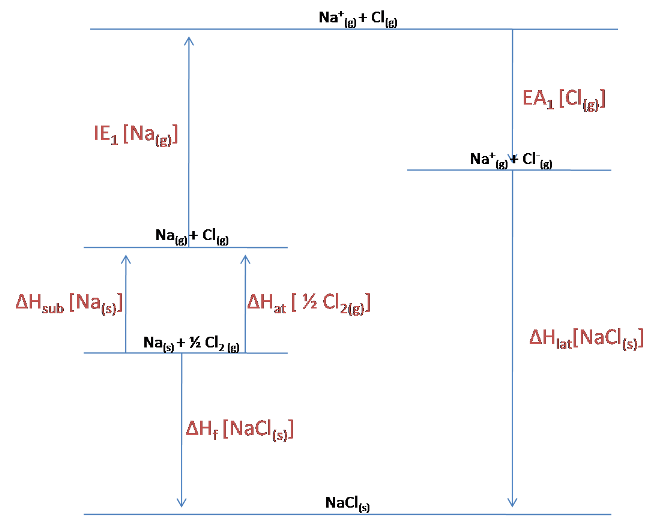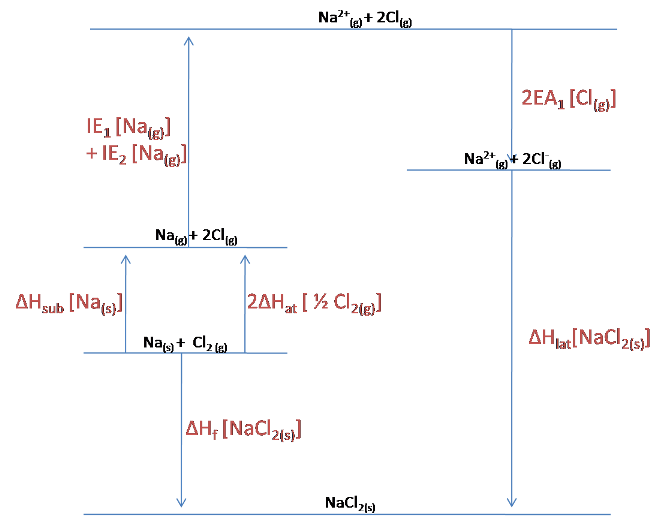#### You may also like### Mathematical Issues for Chemists

A brief outline of the mathematical issues faced by chemistry students.### Reaction Rates

Explore the possibilities for reaction rates versus concentrations with this non-linear differential equation### Catalyse That!

Can you work out how to produce the right amount of chemical in a temperature-dependent reaction?

# What Salt?

##### Age 16 to 18Challenge Level

The Born-Haber cycle for NaCl is shown below:As can be seen from the diagram:

$$\Delta H_{lat}[NaCl_{(s)}] = -EA_1[Cl_{(g)}] - IE_1[NA_{(g)}] -\Delta H_{sub}[Na_{(s)}] -\Delta H_{at}[\frac{1}{2}Cl_{2\ (g)}] + \Delta H_f [NaCl_{(s)}]$$
$$= 349 -496 -108-121 -411$$
$$= -787\ kJ/mol$$

For NaCl$_2$, the following Born-Haber cycle can be constructed:The lattice enthalpy is already given in the question, but we can still use the cycle to calculate the enthalpy of formation of NaCl$_2$.

$$\Delta H_f [NaCl_{2\ (s)}] = \Delta H_{sub}[Na_{(s)}] + 2\Delta H_{at}[\frac{1}{2}Cl_{2\ (s)}] + IE_1[Na_{(g)}] + IE_2[Na_{(g)}] + 2EA_1[Cl_{(g)}] + \Delta H_{lat}[NaCl_{2\ (s)}]$$
$$= 108 + 2(121) + 496 + 4563 +2(-349) +(-3360)$$
$$= +1351\ kJ/mol$$

As this calculation reveals, the enthalpy of formation of NaCl$_2$ is actually endothermic (positive) rather than endothermic (negative), and so the drawn Born-Haber cycle is actually incorrect. The cycle should reveal that the lattice is higher in energy than the elements that it is formed from.

It is clear to see that NaCl$_2$ actually requires energy to be formed from its constituent elements, whereas NaCl releases energy upon its formation. This is the main reason why NaCl is formed preferentially over NaCl$_2$, since it is lower in energy.

The main contribution that makes the formation of NaCl$_2$ so unfavourable is the large second ionisation energy of sodium. The excessive amount of energy that needs to be put in to remove an electron from an already positively-charged ion means that the overall formation of the lattice is not favourable. Because subsequent ionisation energies are even higher (which are not compensated for by the increased attraction in the lattice), it is likely that the formation of other NaCl$_n$ lattices are even more unlikely.

Simply by plugging the correct ionic radii and born exponents into the Born-Lande equation should yield the results shown below. Alongside are the lattice enthalpies calculated experimentally, for comparison:It can be seen that the Born-Lande equation gives a reasonable approximation for the smaller of the halides, but that as the halide size increases, the discrepancy between theoretical and experimental lattice enthalpies changes. It should be noted foremost that the theoretical value is always lower than the experimental, since it relies solely on electrostatic interactions: there are of course additional covalent forces which strengthen the lattice. The increasing discrepancy for increasing size of halide indicates that the larger halides have an increased amount of covalent bonding, which is not taken into account by the Born-Lande equation. Covalent bonding is the sharing of electrons, and it can be rationalised that increased covalent character is seen for the larger halides, since these halides are more diffuse and so have more polarisable electron densities.